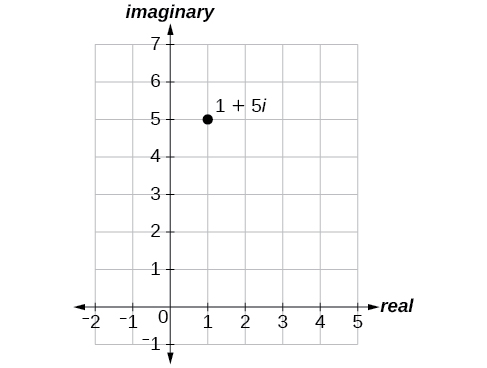# 10.5 Polar form of complex numbers

 Page 1 / 8
In this section, you will:
• Plot complex numbers in the complex plane.
• Find the absolute value of a complex number.
• Write complex numbers in polar form.
• Convert a complex number from polar to rectangular form.
• Find products of complex numbers in polar form.
• Find quotients of complex numbers in polar form.
• Find powers of complex numbers in polar form.
• Find roots of complex numbers in polar form.

“God made the integers; all else is the work of man.” This rather famous quote by nineteenth-century German mathematician Leopold Kronecker sets the stage for this section on the polar form of a complex number. Complex numbers were invented by people and represent over a thousand years of continuous investigation and struggle by mathematicians such as Pythagoras , Descartes , De Moivre, Euler , Gauss , and others. Complex numbers answered questions that for centuries had puzzled the greatest minds in science.

We first encountered complex numbers in Complex Numbers . In this section, we will focus on the mechanics of working with complex numbers: translation of complex numbers from polar form to rectangular form and vice versa, interpretation of complex numbers in the scheme of applications, and application of De Moivre’s Theorem.

## Plotting complex numbers in the complex plane

Plotting a complex number     $\text{\hspace{0.17em}}a+bi\text{\hspace{0.17em}}$ is similar to plotting a real number, except that the horizontal axis represents the real part of the number, $\text{\hspace{0.17em}}a,\text{\hspace{0.17em}}$ and the vertical axis represents the imaginary part of the number, $\text{\hspace{0.17em}}bi.$

Given a complex number $\text{\hspace{0.17em}}a+bi,\text{\hspace{0.17em}}$ plot it in the complex plane.

1. Label the horizontal axis as the real axis and the vertical axis as the imaginary axis.
2. Plot the point in the complex plane by moving $\text{\hspace{0.17em}}a\text{\hspace{0.17em}}$ units in the horizontal direction and $\text{\hspace{0.17em}}b\text{\hspace{0.17em}}$ units in the vertical direction.

## Plotting a complex number in the complex plane

Plot the complex number $\text{\hspace{0.17em}}2-3i\text{\hspace{0.17em}}$ in the complex plane    .

From the origin, move two units in the positive horizontal direction and three units in the negative vertical direction. See [link] .

Plot the point $\text{\hspace{0.17em}}1+5i\text{\hspace{0.17em}}$ in the complex plane.## Finding the absolute value of a complex number

The first step toward working with a complex number in polar form is to find the absolute value. The absolute value of a complex number is the same as its magnitude , or $\text{\hspace{0.17em}}|z|.\text{\hspace{0.17em}}$ It measures the distance from the origin to a point in the plane. For example, the graph of $\text{\hspace{0.17em}}z=2+4i,\text{\hspace{0.17em}}$ in [link] , shows $\text{\hspace{0.17em}}|z|.$

## Absolute value of a complex number

Given $\text{\hspace{0.17em}}z=x+yi,\text{\hspace{0.17em}}$ a complex number, the absolute value of $\text{\hspace{0.17em}}z\text{\hspace{0.17em}}$ is defined as

$|z|=\sqrt{{x}^{2}+{y}^{2}}$

It is the distance from the origin to the point $\text{\hspace{0.17em}}\left(x,y\right).$

Notice that the absolute value of a real number gives the distance of the number from 0, while the absolute value of a complex number gives the distance of the number from the origin,

## Finding the absolute value of a complex number with a radical

Find the absolute value of $\text{\hspace{0.17em}}z=\sqrt{5}-i.$

Using the formula, we have

$\begin{array}{l}|z|=\sqrt{{x}^{2}+{y}^{2}}\hfill \\ |z|=\sqrt{{\left(\sqrt{5}\right)}^{2}+{\left(-1\right)}^{2}}\hfill \\ |z|=\sqrt{5+1}\hfill \\ |z|=\sqrt{6}\hfill \end{array}$

Find the absolute value of the complex number $\text{\hspace{0.17em}}z=12-5i.$

13

## Finding the absolute value of a complex number

Given $\text{\hspace{0.17em}}z=3-4i,\text{\hspace{0.17em}}$ find $\text{\hspace{0.17em}}|z|.$

Using the formula, we have

$\begin{array}{l}|z|=\sqrt{{x}^{2}+{y}^{2}}\hfill \\ |z|=\sqrt{{\left(3\right)}^{2}+{\left(-4\right)}^{2}}\hfill \\ |z|=\sqrt{9+16}\hfill \\ \begin{array}{l}|z|=\sqrt{25}\\ |z|=5\end{array}\hfill \end{array}$

The absolute value $\text{\hspace{0.17em}}z\text{\hspace{0.17em}}$ is 5. See [link] .

the third and the seventh terms of a G.P are 81 and 16, find the first and fifth terms.
if a=3, b =4 and c=5 find the six trigonometric value sin
pls how do I factorize x⁴+x³-7x²-x+6=0
in a function the input value is called
how do I test for values on the number line
if a=4 b=4 then a+b=
a+b+2ab
Kin
commulative principle
a+b= 4+4=8
Mimi
If a=4 and b=4 then we add the value of a and b i.e a+b=4+4=8.
Tariq
what are examples of natural number
an equation for the line that goes through the point (-1,12) and has a slope of 2,3
3y=-9x+25
Ishaq
show that the set of natural numberdoes not from agroup with addition or multiplication butit forms aseni group with respect toaaddition as well as multiplication
x^20+x^15+x^10+x^5/x^2+1
evaluate each algebraic expression. 2x+×_2 if ×=5
if the ratio of the root of ax+bx+c =0, show that (m+1)^2 ac =b^2m
By the definition, is such that 0!=1.why?
(1+cosA+IsinA)(1+cosB+isinB)/(cos@+isin@)(cos$+isin$)
hatdog
Mark
jaks
Ryan
how we can draw three triangles of distinctly different shapes. All the angles will be cutt off each triangle and placed side by side with vertices touching

#### Get Jobilize Job Search Mobile App in your pocket Now!ByBy P. Wynn NormanBy OpenStaxBy Royalle MooreBy Brooke DelaneyBy Edward BitonBy OpenStaxBy OpenStaxBy Richley CrapoBy Jordon HumphreysBy OpenStax